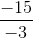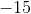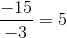# GED Math : Operations with Negative Numbers

## Example Questions

1 2 3 4 6 Next →

### Example Question #161 : Basic Operations

Solve the equation:Explanation:

Dividing with a negative is the same as multiplying with a negative. You only have the number be negative if the one of the numbers is negative and the other is positive. If both numbers are negative, then they will cancel each other and be positive.

Here we can see that both of our numbers are negative,and. Because of this we won't add a negative sign in our answer because both will cancel each other.

Divide the problem as you would, making sure to leave out the negative sign.Our final answer is.

1 2 3 4 6 Next →

### All GED Math Resources